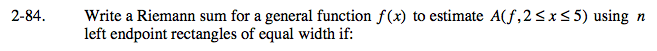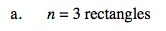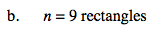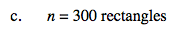### Home > CALC > Chapter 2 > Lesson 2.2.3 > Problem2-84

2-84.
1. Write a Riemann sum for a general function f(x) to estimate A(f, 2 ≤ x ≤ 5) using n left endpoint rectangles of equal width if: Homework Help ✎

1. n = 3 rectangles

2. n = 9 rectangles

3. n = 300 rectanglesGeneral form of left-endpoint Riemann Sum:

n represents number of rectangles
a represents starting value on the x-axis
b represents ending value on the x-axis